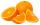# Expression of a variable from the formula + prime numbers - math problems

#### Number of problems found: 8

• QuotientFind quotient before the bracket - the largest divisor 51 a + 34 b + 68 121y-99z-33
• OrangesMother divided her three children's oranges in a ratio of 6:5:4. Two children gave 45 oranges. How many oranges were there?
• Bus linesBuses connections are started from bus stop on its regular circuit as follows: No. 27 bus every 27 minutes and No.18 every half hour. What time started this two bus lines run if the bus stop met at 10:15 am.?
• An exampleAn example is playfully for grade 6 from Math and I don't know how to explain it to my daughter when I don't want to use the calculator to calculate the cube root. Thus: A cuboid was made from a block of 16x18x48 mm of modeline. What will be the edge of t
• PagansElena cut out same circle-shaped pagans and put them on a rectangular sheet so that the neighboring pagans were touching each other and the pagans were touching the walls of the sheet on the edges. Each pagans occupied 28.26 cm2 of the bottom of the sheet
• Cuboid wallsCalculate the cuboid volume if its different walls have an area of 195cm², 135cm², and 117cm².
• Minimum surfaceFind the length, breadth, and height of the cuboid-shaped box with a minimum surface area, into which 50 cuboid-shaped blocks, each with length, breadth, and height equal to 4 cm, 3 cm, and 2 cm respectively, can be packed.
• Profitable companyThree businessman decide to open up their own company. They agree to distribute the yearly profits made in the same ratio as their initial investments. They invest R 50 000, R 75 000 and R25 000, respectively. The profit made by the company in the first y

We apologize, but in this category are not a lot of examples.
Do you have an interesting mathematical word problem that you can't solve it? Submit a math problem, and we can try to solve it.

We will send a solution to your e-mail address. Solved examples are also published here. Please enter the e-mail correctly and check whether you don't have a full mailbox.

Please do not submit problems from current active competitions such as Mathematical Olympiad, correspondence seminars etc...

Expression of a variable from the formula - math word problems. Prime numbers - math word problems.